Get inspired by the success stories of our students in IIT JAM MS, ISI  MStat, CMI MSc DS.  Learn More

# ISI MStat 2018 PSA Problem 13 | Probability of functionsThis is a beautiful problem from ISI MStat 2018 PSA problem 13 based on probability of functions. We provide sequential hints so that you can try .

## Probability - ISI MStat Year 2018 PSA Problem 13

Consider the set of all functions from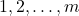to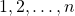where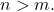If a function is chosen from this set at random, what is the probability that it will be strictly increasing?

•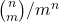••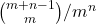•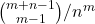### Key Concepts

combination

Answer: isISI MStat 2018 PSA Problem 13

A First Course in Probability by Sheldon Ross

## Try with Hints

What is the total number of functions fromtowhere (n>m)

You have to choose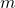numbers amongand assign it to theFor each element of, there are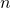options from.
Hencenumber of functions .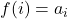The number of ways to select Selectelements amongis the same as the number of strictly ascending subsequences of length m taken from 1, 2, 3, ..., n, which is the same as the number of subsets of size m taken from {1,2,3,…,n}, which is.

Hence the probability that it will be strictly increasing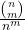## Subscribe to Cheenta at Youtube

This is a beautiful problem from ISI MStat 2018 PSA problem 13 based on probability of functions. We provide sequential hints so that you can try .

## Probability - ISI MStat Year 2018 PSA Problem 13

Consider the set of all functions fromtowhereIf a function is chosen from this set at random, what is the probability that it will be strictly increasing?

••••### Key Concepts

combination

Answer: isISI MStat 2018 PSA Problem 13

A First Course in Probability by Sheldon Ross

## Try with Hints

What is the total number of functions fromtowhere (n>m)

You have to choosenumbers amongand assign it to theFor each element of, there areoptions from.
Hencenumber of functions .The number of ways to select Selectelements amongis the same as the number of strictly ascending subsequences of length m taken from 1, 2, 3, ..., n, which is the same as the number of subsets of size m taken from {1,2,3,…,n}, which is.

Hence the probability that it will be strictly increasing## Subscribe to Cheenta at Youtube

This site uses Akismet to reduce spam. Learn how your comment data is processed.

### Knowledge Partner